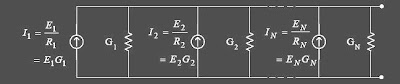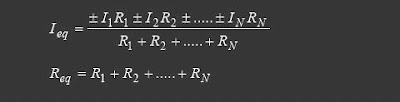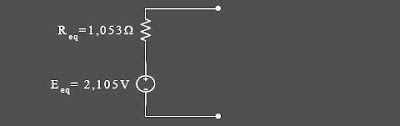## Tuesday, July 21, 2009

### Teorema Millman-Analisis Rangkaian DC

Teorema Millman untuk menganalisis Rangkaian DC

Milman theorems is that a number of series voltage source is connected with the parallel resistor can be simplified to a voltage source only.Steps to apply theorems milman this is as follows:

1. Change all the source currents are a source of tension with the principle of source transformation. So he sets up as follows below2. Determine the total current magnitude Answer algebraic flow from all sources so that there will be a series:3. Change the source of these flows, to be the source voltage by using the principle of source transformation.In general theorems Millman formulatedIf in the series there are a number of sources of electricity flow parallel with the resistor connected in series, then themillman theorems will be :• Example 1
Specify the string Millman, of the following series!specify millman first strand, which is connected with the resistor R3So that the series is to be:• Example 2.
By using the theorems Millman, specify the current that flows in R3!